Return to Contents

## Files Systems, Physical View (Disk Allocation Methods)

• Reference: SGG, Chapter 12, with emphasis on Section 12.4

Examples of File Systems

`man 4 df_ufs`
You can see info about the current state of the file system with
`df -g`
• XFS: A 64-bit high performance journaling filesystem used by IRIX for disks. IRIX is Silicon Graphics' version of UNIX.
• FAT: DOS standard file system, also available on OS/2 and Windows
• FAT32: newer version of FAT with 32 bit block numbers (can address up to 4+ billion blocks)
• NTFS: Windows NT file system, also available on Windows 2000
• High Sierra: CD-ROM file system, standardized on most CD-ROMs.
• NFS: Network file system: makes file systems available to other machines on UNIX. Not a file system in the same sense as the others.

Disk

• a block address is simply an integer telling which one of the blocks it is
• a block address is also called a block number
• usually each block address corresponds to exactly one sector id, but sometimes a block address corresponds to multiple sectors ids.
• I/O layer of the OS translates disk addresses, expressed as a combination of a drive #, a cyclinder #, a track #, and a sector #, into sector ids (and if necessary block numbers).
• use disk for:
• file system
• swap space (managed as part of the file system on UNIX)
• general problem: how do we organize the space on disk?

Disk Allocation Methods

a)
contiguous allocation
• each file occupies a set of consecutive addresses on disk
• each directory entry contains:
• file name
• starting address of the first block
• block address = sector id (e.g., block = 4K)
• length in blocks
• usual dynamic storage allocation problem
• use first fit, best fit, or worst fit algorithms to manage storage
• if the file can increase in size, either
• leave no extra space, and copy the file elsewhere if it expands
• leave extra space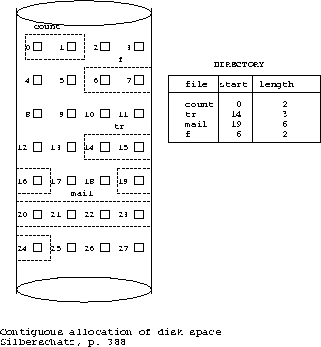b)
• each data block contains the block address of the next block in the file
• each directory entry contains:
• file name
• block address: pointer to the first block
• sometimes, also have a pointer to the last block (adding to the end of the file is much faster using this pointer)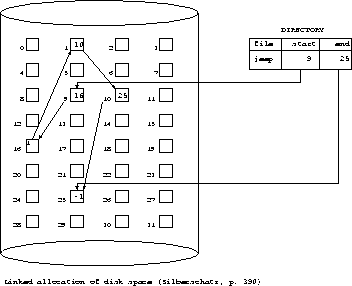• a view of the linked list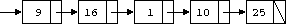c)
indexed allocation
• store all pointers together in an index table
• the index table is stored in several index blocks
• assume index table has been loaded into main memory

i)
all files in one index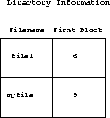The index has one entry for each block on disk.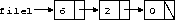• better than linked allocation if we want to seek a particular offset of a file because many links are stored together instead of each one in a separate block
• SGG call this organization a ``linked'' scheme, but I call it an ``indexed'' scheme because an index is kept in main memory.
• problem: index is too large to fit in main memory for large disks
• FAT may get really large and we may need to store FAT on disk, which will increase access time
• e.g., 500 Mb disk with 1 Kb blocks = 4 bytes * 500 K = 2Mb entries
ii)
separate index for each file

• index block gives pointers to data blocks which can be scattered
• direct access (computed offset)
a)
one index block per file (assumes index is contiguous)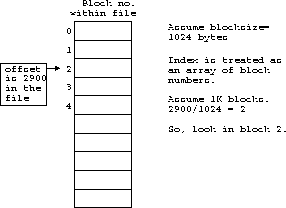b)
linked list of index blocks for each file
c)
multilevel index
d)
combined scheme (i-node scheme) used in UNIX
• described later

Example of Disk Allocation Methods
Question similar to (Exercise 12.1 in SGG or 11.1 in S&G)

Consider a file currently consisting of 150 blocks. Assume that the file control block (and the index block, in the case of indexed allocation) is already in memory. Calculate how many disk I/O operations are requried for continguous, linked, and indexed (single--level) allocation strategies, if, for one block, the following conditions hold. In the contiguous--allocation case, assume that there is no room to grow in the beginning, but there is room to grow in the end. Assume that the block information to be added is stored in memory.

Assumptions:

• Each I/O operation reads or writes a whole block.

• For linked allocation, a file allocation table (FAT) is not used, i.e., only the address of the starting block is in memory.

• The blocks are numbered 1 to 150 and the current positions of these blocks are also numbered 1 to 150.

• All preparation of a block (including putting in the data and any link value) is done in main memory and then the block is written to disk with one write operation.

• The file control block does not have to be written to disk after a change (this is typical where many operations are performed on a file).

• At most one index block is required per file and it does not have to be written to disk after a change.

• For linked allocation, assume that no I/O operations are necessary to add a freed block to the free list.

a)
The block is added in the middle:

Contiguous: Assume that in the middle means after block 75 and before block 76. We move the last 75 blocks down one position and then write in the new block.I/O operations

Linked: We cannot find block 75 without traversing the linked list stored in the first 74 data blocks. So, we first read through these 74 blocks. Then we read block 75, copy its link into the new block (in main memory), update block 75's link to point to the new block, write out block 75, write new block.

74r + 1r + 1w + 1w = 75r + 2w = 77 I/O operations
block75 new

Indexed: Update the index in main memory. Write the new block.

1w = 1 I/O operation

b)
The block is removed from the beginning.

Contiguous: Simply change the starting address to 2.

0 I/O operations

1r = 1 I/O operation

Indexed: Simply remove the block's address from the linked list in the index block.

0 I/O operations

Example of Logical-to-Physical Address Mapping for File Systems

Question:

Consider a file system on a disk that has both logical and physical block sizes of 512 bytes. Assume that the information about each file is already in memory. For the contiguous strategy, answer these questions:

a)
How is the logical-to-physical address mapping accomplished in this system? (For the indexed allocation, assume that a file is always less than 512 blocks long.)

b)
If we are currently at logical block 10 (the last block accessed was block 10) and want to access logical block 4, how many physical blocks must be read from the disk?

Assumptions: 1. Let L be the logical address and let P be the physical address. 2. The assumption in part (a) is poorly given. It's more reasonable to simply assume that the index is small enough to fit into a single block. In fact, a 512 block file will probably require more than a single 512 byte block because block addresses typically require 3-4 bytes each.

(a) Overview The CPU generates a logical address L (a relative offset in a file) and the file system has to convert it to a physical address P (a disk address represented by a block number PB and an offset in this block). For convenience of calculation, we assume that blocks are numbered from 0. In any approach, we can determine the logical block number LB by dividing the logical address L by the logical block size (here 512). Similarly, the offset, which will be the same for logical and physical addresses since the block sizes are identical, is determined by applying modulus. The offset is the same in all approaches.

```        LB := L div 512
offset :=  L mod 512
```

Contiguous: Assume S is the starting address of the contiguous segment. Then a simple approach to mapping the address is:

```        P = S + L
```

If we prefer to consider the block level,

```        PB = SB + LB
```

(b) If we are currently at logical block 10 and we want to access logical block 4 ...

Contiguous: We simply move the disk head back by 6 blocks (from physical block 10 to physical block 4) because the space allocated to the file is contiguous. Then we read block 4, for a total of one read.

Free Space Management
• Bit Vector:
• Each block is represented by 1 bit.
• If a block is free, then the bit is 1, but if the block is in use, then the bit is 0.
• For example, if the disk had 10 blocks, and blocks 2, 4, 5, and 8 were free, while blocks 0, 1, 3, 6, 7, and 9 were in use, the bit vector would be represented as: 0010110010
• Correction to 5th edition of S\&G.
• Free List (Linked List of Free Blocks)
• The address (block number) of the first free block is kept in a designated place in memory.
• The first free block contains a pointer to the next free block, it contains a pointer to the next free block, and so forth.
• Can add a free block to the beginning of the free list in O(1) time.
• Can remove a free block from the beginning of the free list in O(1) time.

Example File System: Original UNIX File System
• layout of a disk containing one UNIX file system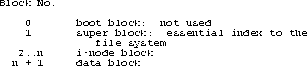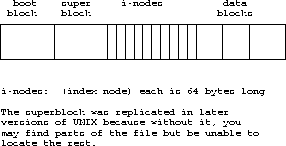The super block contains:

• number of i-nodes
• number of data blocks
• start of the list of free blocks
• first few hundred entries
• the rest of the free list is stored in a block that is otherwise free
Example on UNIX:
df = disk free
df -i /u
/u is the directory on hercules is where student files are stored

```Filesystem    Type   blocks       use     avail  %use     iuse    ifree  %iuse  Mounted
/dev/dsk/dks   efs  7654152   2790059   4864093   36%   158252   647230    20%   /u
```

```
7654152 Kb = total space

2790059 Kb = being used

4864093 Kb = free

i use = no. of i-nodes in use = no. of files

i free = extra i-nodes

```

Each i-node:

• describes one file
• accounting info (owner and protection bits)
• provides the address information for all blocks in the file
• direct pointers to the first 10 blocks
• indirect pointer to a block containing more pointers
• double indirect pointer to blocks of pointers
• triple indirect
• a block of pointers to blocks of pointers to blocks of pointers to data blocks

Sample calculation of maximum file size

• Assume that there are 10 direct pointers to data blocks, 1 indirect pointer, 1 double indirect pointer, and 1 triple indirect pointer
• Assume that the size of the data blocks is 1024 bytes = 1Kb, i.e., BlockSize = 1Kb
• Assume that the block numbers are represented as 4 byte unsigned integers, i.e., BlockNumberSize = 4b
• Some data blocks are used as index blocks. They store 1024 bytes / 4 bytes/entry = 256 entries
• Maximum number of bytes addressed by 10 direct pointers is
```	= Number of direct pointers * Blocksize
= 10 * 1Kb
= 10Kb
```
• Maximum number of bytes addressed by single indirect pointer is
```	= NumberOfEntries * BlockSize
= (Blocksize / BlockNumberSize) * BlockSize
= (1Kb / 4b) * 1Kb
= 256 * 1Kb
= 256Kb
```
• Maximum number of bytes addressed by double indirect pointer is
```	= NumberOfEntries^2 * BlockSize
= (Blocksize / BlockNumberSize)^2 * BlockSize
= (1Kb / 4b)^2 * 1Kb
= (2^10 / 2^2)^2 * (2^10b)
= (2^8)^2 * (2^10)b
= (2^16) * (2^10)b
= 2^6 * 2^20 b
= 64 Mb
```
• Maximum number of bytes addressed by triple indirect pointer is
```	= NumberOfEntries^3 * BlockSize
= (Blocksize / BlockNumberSize)^3 * BlockSize
= (1Kb / 4b)^3 * 1Kb
= (2^10 / 2^2)^3 * (2^10b)
= (2^8)^3 * (2^10)b
= (2^24) * (2^10)b
= 2^4 * 2^30 b
= 16 Gb
```
• Maximum file size is 16Gb + 64Mb + 266Kb

I-Node Organization
• Reference: see p. 165 Tanenbaum handoutReturn to Contents